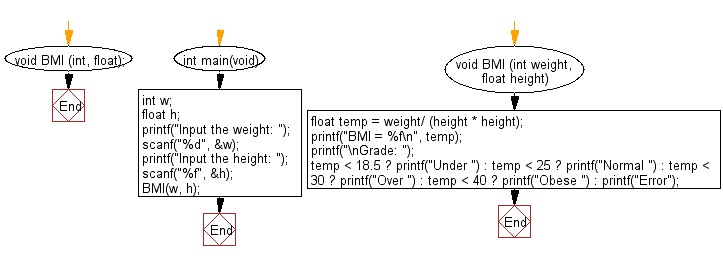﻿ C Program: Calculate body mass index and display the grade - w3resource

# C Exercises: Calculate body mass index and display the grade

## C Basic Declarations and Expressions: Exercise-94 with Solution

Write a C program to calculate body mass index and display the grade from the following grades:
Underweight - BMI < 18.5
Normal weight - 18.5 <= BMI < 25.0
Overweight - 25.0 <= BMI < 30.0
Obesity - 30.0 <= BMI
Where weight is taken in kilograms and height in meters.
Body Mass Index (or BMI) is calculated as your weight (in kilograms) divided by the square of your height (in metres) or BMI = Kg/M2.

Sample Solution:

C Code:

``````#include <stdio.h>

// Function to calculate and display BMI
void BMI(int, float);

int main(void) {
int w; // Variable to store weight
float h; // Variable to store height

// Prompt user for weight and height
printf("Input the weight: ");
scanf("%d", &w);
printf("Input the height: ");
scanf("%f", &h);

// Call the BMI function with provided weight and height
BMI(w, h);
}

// Function to calculate and display BMI
void BMI(int weight, float height) {
// Calculate BMI using the provided formula
float temp = weight / (height * height);

// Display the calculated BMI
printf("BMI = %f\n", temp);

// Determine BMI grade and display it
temp< 18.5 ? printf("Under ") : temp < 25 ? printf("Normal ") : temp < 30 ? printf("Over ") : temp < 40 ? printf("Obese ") : printf("Error");
}

``````

Sample Output:

```Input the weight: 65
Input the height: 5.6
BMI = 2.072704

```

Flowchart:C programming Code Editor:

What is the difficulty level of this exercise?

Test your Programming skills with w3resource's quiz.

﻿